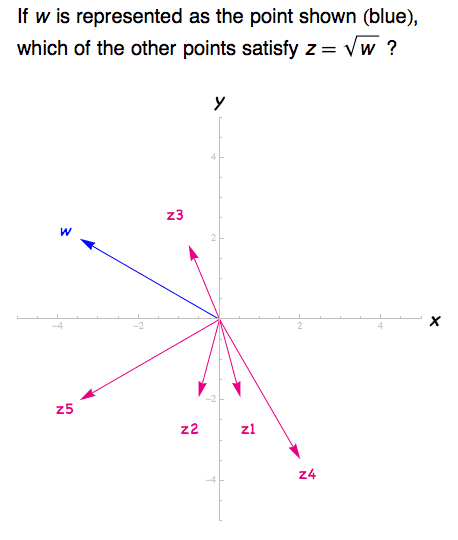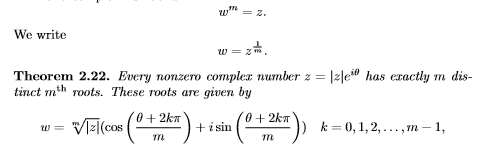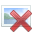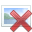# Complex Plane - Graphing Powers

• zr95

## Homework Statement

If W is represented as the point shown in blue which of the other points satisfy z=Sqrt[w]?## Homework Equations## The Attempt at a Solution

I'm trying to study for a test and this is a practice problem and the book doesn't go into great detail about this sort of problem graphically but I think I have it figured out, just maybe not the best way of going about it.

Because the power is 1/2 I imagine that means there are 2 Square roots of the complex number. Each varying by 2*pi

I'm assuming W looks like its at 5pi/6 = 150 degrees just for reference.
150/2=75 degrees
(150+360)/2=255 degrees

I move 75 degrees from the x-axis and there is no line there so I go to my next option.
Then I move 255 degrees off the x-axis and think maybe I hit where Z2 is located. Is my logic correct?

Last edited:
I move 75 degrees from the x-axis and there is no line there
I think you are doing the right thing there, and the proper answer is "none of the other points"!
z2 satisfies ##z^2 = w## but not ## z=\sqrt w##.

## Homework Statement

If W is represented as the point shown in blue which of the other points satisfy z=Sqrt[w]?
View attachment 100202

## Homework Equations

View attachment 100203

## The Attempt at a Solution

I'm trying to study for a test and this is a practice problem and the book doesn't go into great detail about this sort of problem graphically but I think I have it figured out, just maybe not the best way of going about it.

Because the power is 1/2 I imagine that means there are 2 Square roots of the complex number. Each varying by 2*pi *

I'm assuming W looks like its at 5pi/6 = 150 degrees just for reference.
150/2=75 degrees
(150+360)/2=255 degrees

I move 75 degrees from the x-axis and there is no line there so I go to my next option.
Then I move 255 degrees off the x-axis and think maybe I hit where Z2 is located. Is my logic correct?

The statement Which I placed an asterisk next might better read something like:
Because the power is 1/2, that means there are 2 Square roots of the complex number. The square of each differing by 2π.​

Sometimes it's easier to see graphically if you consider that the given vector (or point or complex number) is at some negative angle. In this case that's -210° (i.e. 30° more negative than -180°). Half of that is -105° (15° more negative than -90°)

Generally, check the magnitude of the vector / complex number.

.

The statement Which I placed an asterisk next might better read something like:
Because the power is 1/2, that means there are 2 Square roots of the complex number. The square of each differing by 2π.​

Sometimes it's easier to see graphically if you consider that the given vector (or point or complex number) is at some negative angle. In this case that's -210° (i.e. 30° more negative than -180°). Half of that is -105° (15° more negative than -90°)

Generally, check the magnitude of the vector / complex number.

.
I learned that the range of ##\sqrt z## is the right half of the complex plane only. Math dislikes multiple-valued functions.

I learned that the range of ##\sqrt z## is the right half of the complex plane only. Math dislikes multiple-valued functions.
The correct answer in this problem happens to be in the left half of the complex plane.

Same way as in real numbers ##\sqrt 4 = -2 ## ?

Same way as in real numbers ##\sqrt 4 = -2 ## ?
Oh! You're referring to the principle square root for real numbers. Yes, in that case ##\ \sqrt{4}=2 \ ##.

It's my experience, that in the context of complex numbers, while a principle branch is often defined for roots of a complex numbers, that definition is not necessarily universally accepted.

It's quite common to consider that every non-zero complex number has two square roots, three cube roots, four fourth roots, etc.

Oh! You're referring to the principle square root for real numbers. Yes, in that case ##\ \sqrt{4}=2 \ ##.

It's my experience, that in the context of complex numbers, while a principle branch is often defined for roots of a complex numbers, that definition is not necessarily universally accepted.

It's quite common to consider that every non-zero complex number has two square roots, three cube roots, four fourth roots, etc.
I'm with BvU on this. There can be multiple "square roots", but the √ symbol should represent the (complex) square root function, which is defined to have a result with argument θ, where -π/2<θ<=π\2.

In brief, as a matter of principle, √ always gives the principal square root.We're not going to turn math into a democracy where the majority is right, I hope? Isn't there a Big Book or something we can consult ?My two cents is that if for complex numbers ##\sqrt 4## can be ##-2## then the discrepancy with ##\sqrt 4 \ne -2## for real numbers constitutes a nasty breach of something. After all, real numbers should be a decently behaving subset of complex numbers. (Drat, what's the ##LaTeX## symbol for ##\mathbb R##)

Last edited:
We're not going to turn math into a democracy where the majority is right, I hope?
This isn't a question of being right or wrong mathematically, but only a question of agreeing on definitions. Even if there is a Big Book for that, it is some kind of democratic process that leads to its contents. Anyway, I did check my understanding at https://en.wikipedia.org/wiki/Square_root#Square_roots_of_negative_and_complex_numbers and http://mathworld.wolfram.com/SquareRoot.html. The latter says, e.g., "The principal square root of a numberis denoted [PLAIN]http://mathworld.wolfram.com/images/equations/SquareRoot/Inline24.gif" [Broken]

Last edited by a moderator:
•BvU
While you're all discussing whatever it is you're discussing - you lost me at #2 - can I ask you to spare a comment to extend my understanding, if that's not a hopeless ask?
I thought I understood the OP and just watched to see if anyone had any better ideas than me.
Then post #2 said
z2 satisfies ##z^2 = w## but not ## z=\sqrt w##.
I was under the apparently mistaken impression that this was the definition of square root. For example, from Wolfram, "A square root ofis a numbersuch that [PLAIN]http://mathworld.wolfram.com/images/equations/SquareRoot/Inline3.gif." [Broken] So what am I missing here? (The Wolfram article is talking reals here, but it does go on to discuss complex roots without seeming to make any change to the definition.)

Then while I was struggling with that,
... Because the power is 1/2, that means there are 2 Square roots of the complex number. The square of each differing by 2π.
Assuming we are talking about θ in ## z = \lvert {z} \rvert e^{iθ} ## how can it "differ" by 2π ?
Is that like saying 2, 1x2, 1x1x2 differ by 0? I always thought ## e^{iθ} = e^{iθ +2nπ }## not that they differed at all?

And just a non-substantive btw point, "... Math dislikes multiple-valued functions." from BvU, I was taught at secondary school, that functions gave unique values, else they are not functions. So sqrt is not a function, unless you are talking of the principal value sqrt. (I think they might have been relations? But my memory is less clear on that point.)

Last edited by a moderator:
"A square root ofis a numbersuch that." So what am I missing here?
As I wrote in post #8, "a square root of z" is different from "√z". √z is a well defined function, and therefore has only one value for each input. It is defined for all complex z=re (r>=0, -π<θ<=π) to return (√r)ei(θ/2) where √r is the non-negative square root of the non-negative real r .

I agree it's a convention - but a pretty strict one, that is part of a larger set (and I bet all programming languages adhere). I always learned that ##x^2 = 1## (in ##\mathbb R##) has two solutions and that ##\sqrt1=1## and not ##\pm 1##. And until this day I never had the term 'principal square root' in mind. Lucky me - good teachers.

I find the Wolfram description lacking. 'has two square roots' , 'the other square root', 'generally taken' .

@zr95: I hope you don't feel this thread has been hijacked. Discuss it with your teacher. He (she) just might consider changing the exercise to avois this kind of complication. If he/she's really devious the exercise could ask for 'a square root of w', but didactically it would be a lot better to add an arrow at ##-##z2.

@all: good thread -- sets they grey cells at work !

Last edited:
Because the power is 1/2 I imagine that means there are 2 Square roots of the complex number. Each varying by 2*pi
I would say, there are two roots differing by pi. (Not by 2*pi. )
In general for nth roots they differ by 2π/n and one root is at θ/n

I think you should also take account of the magnitude (z2 is about right in fact) because a number might have the correct angle, but the wrong magnitude. Since magnitudes are all positive, you simply need ## \lvert z \rvert = \lvert\ √(\lvert w \rvert) \rvert ## (written to try to avoid attacks by pedants, rather than for clarity!)

I think your method is fine and can't be improved, other than making a rough estimate based on visually n-secting the angle and noting the n roots are equally spaced around the circle.

would say, there are two roots differing by p
Yes, but only one of them is √w. The other is -√w. The z2 shown could be -√w.

•BvU
...
Then while I was struggling with that,
Assuming we are talking about θ in ## z = \lvert {z} \rvert e^{iθ} ## how can it "differ" by 2π ?
Is that like saying 2, 1x2, 1x1x2 differ by 0? I always thought ## e^{iθ} = e^{iθ +2nπ }## not that they differed at all?
Yes. I should have said something like "their arguments differ by 2π"; in reference to the square of z.

Yes, but only one of them is √w. The other is -√w. The z2 shown could be -√w.
But which is which?! (As a matter of fact, I don't agree with your statement, but let's look at it.)
Say W were say ##7 +24i## for example. Then we find two roots, ## +4 +3i \ \ and \ -4 -3i ## So which of these is ## - \sqrt {W} ## and which ## + \sqrt{W} ## (or ## \sqrt{W}## as you dub it?)
You may favour calling ##-4 -3i## the negative root because you become fixated by the signs, but there really is no reason to do so. Because if instead we took W to be ##7 -24i## then our roots pop out as ##+4 -3i## and ##-4 +3i##.
Now which do you choose for ##\sqrt{W}##? Is it the one with the positive real part or the one with the positive imaginary part? I bet you go for the one with the positive real part again! So we have your rule, the principal root is the one with the positive real part.
So now we look at the roots of -1, which turn out to be ## +0 -1i## and ##-0 +1i## and you have to say ## \sqrt{-1} = -i## and ## i = -\sqrt{-1}## (or if you don't like ±0, then call them both +0, then you have to go on to a second rule to choose the principal root.
But then I move on to cube roots, and if necessary nth roots. How are you going to pick the "real" root when several of them have both parts positive, several both negative, several one of each?

Ok, probably all a spurious load of spheres, but while you can have this notion of a principal root for positive reals and maybe negative reals, it makes no sense to have principal roots for complex numbers. For complex numbers all roots are born free and equal. Is this not self-evident?

Yes. I should have said something like "their arguments differ by 2π"; in reference to the square of z.
I wasn't worried about the "arguments" bit. I'm happy to accept the obvious intent and I only wrote it out explicitly to make sure you realized I'd taken it as intended.
My problem is with the 2π. As far as I can see, square roots differ by 1π not 2π (or you can say, 2π/2). Just as cube roots differ by 2π/3, 4th roots by 2π/4, ... nth roots by 2π/n.

Edit: Ooops. Just spotted my mistake. Correction to follow.

Last edited:
But which is which?! (As a matter of fact, I don't agree with your statement, but let's look at it.)
Say W were say ##7 +24i## for example. Then we find two roots, ## +4 +3i \ \ and \ -4 -3i ## So which of these is ## - \sqrt {W} ## and which ## + \sqrt{W} ## (or ## \sqrt{W}## as you dub it?)
You may favour calling ##-4 -3i## the negative root because you become fixated by the signs, but there really is no reason to do so. Because if instead we took W to be ##7 -24i## then our roots pop out as ##+4 -3i## and ##-4 +3i##.
Now which do you choose for ##\sqrt{W}##? Is it the one with the positive real part or the one with the positive imaginary part? I bet you go for the one with the positive real part again! So we have your rule, the principal root is the one with the positive real part.
So now we look at the roots of -1, which turn out to be ## +0 -1i## and ##-0 +1i## and you have to say ## \sqrt{-1} = -i## and ## i = -\sqrt{-1}## (or if you don't like ±0, then call them both +0, then you have to go on to a second rule to choose the principal root.
But then I move on to cube roots, and if necessary nth roots. How are you going to pick the "real" root when several of them have both parts positive, several both negative, several one of each?

Ok, probably all a spurious load of spheres, but while you can have this notion of a principal root for positive reals and maybe negative reals, it makes no sense to have principal roots for complex numbers. For complex numbers all roots are born free and equal. Is this not self-evident?
I supplied the standard definition of the principal square root in post #8 and links to supporting references in post #10. You might care to search the web for more.

Ooops. I just noticed that you did say, in reference to the square of z." My previous reply is obviously referring to the roots themselves.

So, are you saying that if I take the two square roots of a complex number and square them, I will get answers which differ in their argument by 2π?
So if I take my old friends from #17, ##+4 -3i## and ##-4 +3i## the square roots of ## 7 -24i## and square them, my answers will arguments which differ by 2π?
I get ##(+4-3i)^2 = 7 -24i## and ##(-4+3i)^2 = 7-24i## both of which have exactly the same argument, arctan(24/7). No difference at all.
So perhaps your rule only works if you calculate using polar notation? (And don't simplify your results.)

My problem is probably that I don't use maths at all, just the pictures. Two equal numbers on an Argand diagram are pretty much indistinguishable to my eye.

I supplied the standard definition of the principal square root in post #8 and links to supporting references in post #10. You might care to search the web for more.
Indeed you did and I should have remembered that. I did not look back at #8 when responding to the later comment. Sorry.

The Wolfram reference for Principal Square Root seems to refer to positive real numbers saying, "...The concept of principal square root cannot be extended to real negative numbers ..." .and satisfied, I did not originally look further. But their main entry does indeed go on to mention a principal sqrt for complex numbers. The Wiki article I must confess uses concepts completely unknown to me, so does not help, beyond proving your case.

Probably good advice to search the web, but i don't suppose I'll bother, now that you've told me the answer. I can't think of anything more I want to know about principal roots, except things I probably couldn't understand. I think it will become just one more topic I have to leave to the experts. For myself I'll continue to look at all roots, as I find any of them can be significant. Only real ones seem to be in a class of their own - sometimes.

Let's see now. What was the initial question?If we take the strict interpretation that the problem asks us to use a strict interpretation of ##\ \sqrt{w}\ ## as the principle square root, that is the square root in the right half-plane or on the positive imaginary axis, then none of the vectors is correct.

If we take the problem to mean, "Find ##z##, such that ##\ z^2=w\ ##," then ##\ z_2 \ ## is the solution as OP found.

I was merely trying to give OP an alternate way to find that solution.

Your non-strict interpretation is fine by me. Like you I was looking at how the original question could be answered.

Although I'd never come across the notion of √ z meaning "the principal square root of z", I'm quite happy to believe that some people use it that way.
But if someone told me that, say -2 is not = √4 , I would not ( will not) believe them, irrespective of context, as all my experience tells me that it is. If they say that answer gives a solution outside some boundary condition for a particular problem, fine, but you need to have considered that value to decide.

Reflecting on my own practice I see that, I will normally write, ## (x^2=y)⇒x=±√y ## as a sort of reminder not to lose the other root in my algebraic manipulation, but normally just ## (x^2=2) ⇒ (x=√2) ⇒(x=±1.414)## leaving the two roots implicit until the end in arithmetic.

I can't think of any situation where you'd be better off assuming √ means principal root, than √ means both roots. At worst you end up with ±(±n) for an answer, which is no problem. If you end up with +n only, that could be bad.

I don't know what zr95 thinks, but it seems to me that the more correct answer is not the more helpful answer here.

but merlin can then not give a value for the derivative of any function with a square root somewhere. Very tedious things that way become

Now that's something I hadn't thought of.
Because I don't recall meeting such a problem, I suppose I'd just assumed (or more likely had never given it much thought) that roots only arose in the solution of quadratic (& above) equations, which need more than one solution and may need more than one route to the roots.
I can see how it would have been convenient to convert sqrt into a function by redefining it (or defining it it it was undefined common usage at the time) as the principal root. Obviously I missed that, along with almost everything else in real, serious maths and physics!
Just as I worry about missing solutions if I don't consider all the roots, I suppose there may be situations where it important not to allow a non-principal root (such as the negative sqrt.) The only formula that comes to mind is boatspeed with sqrt of waterline length. If you used the negative root there, presumably the boatspeed would be negative meaning it was going backwards - which would not just be outside the range of consideration, but would invalidate the formula it came from because of the anisotropic shape of boat hulls. I guess you've convinced me to give up the habits of (a lifetime - 13 years). But I expect I'll still think about all those other poor little roots before casting them out into the cold